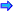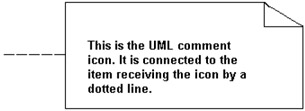# Introduction to UML Style of Design

Today's programming is based more and more each day on classes and object oriented programming (OOP). In addition to C++ and Java, the new language C# will be a major tool in the creation of Internet programming as well as desktop programming. The tools of the past: pseudo code, structure charts and flowcharts were great tools for structured programming but new tools are needed that better support object oriented design (OOD). One of these tools is the UML (Unified Modeling Language).

What you should do is go onto the Internet and study UML by researching some of the companies, individuals and organizations involved in UML. The discussion in these lectures shall only be an introduction to the topic. If you intend to continue your study of C++, Java or C#, it is advisable that you explore this topic further. There are several books out on the market. I would recommend that after studying these lectures you buy one and study this topic further. In addition, if you can arrange it, I would recommend that you take a course on OOD.

Another reason to study UML is that there are companies who have designed software that will take a program design in UML 2.1+ and write the corresponding C++ code. In addition there are programs on the market that will take C++ programs and write the UML design documents.

In this lecture the concept of class was introduced. This construct consists of data members (sometimes called attributes) and member functions (sometimes called operators or methods). A class is a template that acts as a pattern for the objects of the class. It may be visualized as a cookie cutter for molding cookies i.e. the objects. To create design models of a class, the UML class icon is used. It consists of a rectangle with three sub rectangles (there may be more than three as discussed below). Above you saw an example of a C++ program that was based on the class Date. See dateclas.cpp. The UML class icon for the class Date would be represented as in the following:

Date

-month:integer

-day:integer

-year:integer

+setDate():void

+showMonthName():void

The first sub rectangle contains the name of the class (additional information may also appear as you will see later). Notice in the second compartment of the rectangles above that each data member is listed followed by a colon followed by its data type. In the bottom compartment are listed the two member functions from the class Date. The output of these methods are listed after the method header separated by a colon. Notice also the minus signs (which mean that the members are private) and the plus signs (which mean the members are public.) These signs appear for both the data members and the member functions.

The data members of the class Date have restrictions on them other than their data type. For example, the data member month may only have the values from 1 to 12. The data member day may only have the values from 1 to 31 and in addition the day will depend on the value of month for the particular object. These member restrictions can be indicated in the UML class icon in the following manner:Open table as spreadsheet

Date

-month:integer

<< month = 1 to 12>>

-day:integer

<< day = 1 to 31 depending on the value of

-year:integer

month>>

+setDate():void

+showMonthName():void

To denote the different access sections for the data members and member functions, you could use the UML stereotypes in the class rather than the plus and minus listed above. The stereotypes are indicated by placing what would look like comments above groups of data members or member functions as in the following:

Date

<<private>>

month:integer

day:integer

year:integer

<<public>>

setDate()

showMonthName()

Additional stereotypes may be added to these rectangles to better describe the role of groups of the attributes and groups of the methods.

When specifying how the class will be used, an additional rectangle may be added to the bottom of the above rectangle. In this bottom rectangle would be a description of the roll that the class plays in the programs. This is referred to as the class responsibility as in the following example:

Date

<<private>>

month:integer

day:integer

year:integer

<<public>>

setDate()\

showMonthName()

This class is used to model the use of dates in any program.

In the example dateclas.cpp above, an object invoiceDate of the class Date was defined. To represent this object, a UML object icon could be used. The object icon is a rectangle with the object's name in it followed by a colon followed by the class name. All of this would be underlined as in the following icon:

 invoiceDate:Date

An object may be a special instance of a class. For example in the Unix operating system, the date 1/1/1970 is a special date from which all other dates are calculated. The UML icon for this Date could be the following:

startDate:Date

month = 1

day = 1

year = 1970

This is the date used in to which all other dates are compared.

Notice in the above icon the use of the object responsibility.

As with flowcharts, there is a need for internal comments with the UML icons and diagrams.In another example above, you considered the class Account. It would be represented by the following UML icon:

Account

-theBalance:float

+setBalance(R float):void

+getBalance():float

+doDeposit(R float):void

+doWithdrawal(R float):void

In this example, notice that each of the methods has an additional notation not seen in the previous example. Notice that the signature is listed in the methods: setBalance(), doDeposit() and doWithdrawal(). In addition the output is listed in getBalance(). The signature appears similar to how it would appear in C++ except the data type follows the variable. The output data type follows the method name and its signature.

Another difference is that instead of placing <<private>> and <<public>> in these rectangles, the symbols + and - are used to denote the same thing. That is if either an attribute or a method are private a - precedes them. If either an attribute or a method is public then a + precedes them as in the above example. The method to represent public and private using + and - is now the preferred way to do it and that is the way Visio represents these properties. If a class has a static attribute then the word static should precede the declaration of the attribute.

In examples you will find in some books on UML design, the complete definition of the function may appear. In other books you will find that instead of this, there will be listed in a pseudo code document the pseudo code description of the methods as we have done previously in the discussion above for functions. This second approach is now the preferred way to represent the logic of methods.